# pytorch模型保存与加载总结

## pytorch模型保存与加载总结

### 模型保存与加载方式

``````model = VGGNet()
torch.save(model.state_dict(), PATH)``````

``````model = VGGNet()
torch.save(model, PATH)``````

``````new_model = VGGNet()

``new_model = torch.load(PATH) ``

### 打包保存tar

``````state = {
'epoch': 10,                            # 保存当前的迭代次数
'state_dict': resnet18.state_dict(),    # 保存模型参数
'learning_rate': 0.000456,              # 保存学习率
}
torch.save(state, 'qqq.pth.tar')``````

``````checkpoint = torch.load('qqq.pth.tar')
epoch = checkpoint['epoch']

### 多卡训练遇到的问题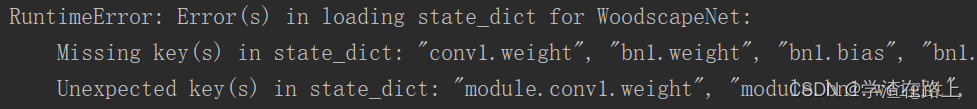``````model = torch.nn.DataParallel(model)
torch.save(model.module.state_dict(), 'fff.pth'))``````

``model.load_state_dict(torch.load(load_pth_name))``

``````model = torch.nn.DataParallel(model)
torch.save(model.state_dict(), 'fff.pth'))``````

``````state_dict = torch.load(load_pth_name)
from collections import OrderedDict
new_state_dict = OrderedDict()
for k, v in state_dict.items():
name = k[7:]  # remove `module.`
new_state_dict[name] = v

OrderedDict

``````from collections import OrderedDict
ordered1 = OrderedDict(a=1, b=2, c=3)
ordered2 = OrderedDict()
print(ordered1)
print(ordered2)
ordered2['bbb'] = 1
ordered2['aaa'] = 2
print(ordered2)``````

``````OrderedDict([('a', 1), ('b', 2), ('c', 3)])
OrderedDict()
OrderedDict([('bbb', 1), ('aaa', 2)])``````

### torch.jit

pytorch为了在C++上能够运行训练好的模型，出了个包叫libtorch。libtorch之后，你就能够用C语言调用pytorch训练好的模型，毕竟最后工程化的东西不可能让你使用python的代码，这种东西其实有挺多的，比如说opencv就能够读取pytorch训练好的模型，然后使用c的代码；英伟达也有tensorRT；什么阿里、小米啥的有mnn、ncnn，都是偏C，偏工程化的东西。

jit 保存模型

``````resnet18 = models.resnet34(pretrained=True)
dummy_input = torch.rand(1, 3, 256, 256).to('cpu')
torch_trace_model = torch.jit.trace(resnet18, dummy_input)
torch.jit.save(torch_trace_model, 'www.pth')``````

jit 保存模型的方式，和之前的保存方式还是有一些差别，jit 需要指定输入的尺寸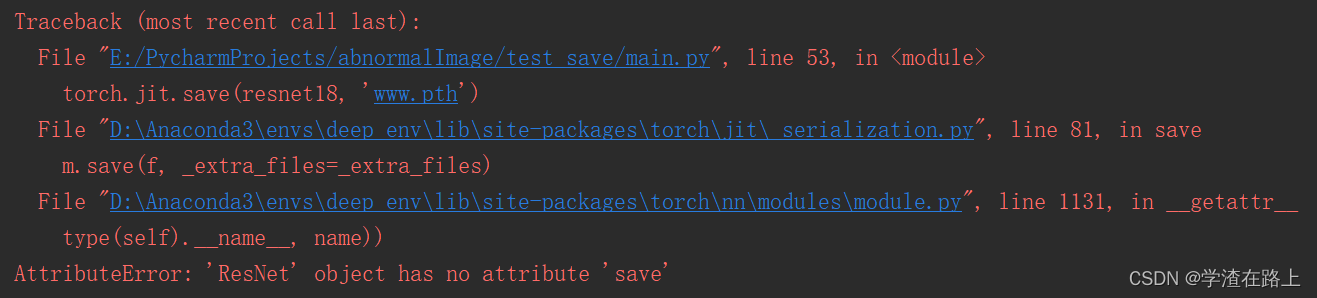trace与script

``````class ControlFlowModule(torch.nn.Module):
def __init__(self):
super(ControlFlowModule, self).__init__()
self.l0 = torch.nn.Linear(4, 2)
self.l1 = torch.nn.Linear(2, 1)
​
def forward(self, input):
if input.dim() > 1:
​
out0 = self.l0(input)
out0_relu = torch.nn.functional.relu(out0)
return self.l1(out0_relu)
​
​
if __name__ == '__main__':
a = torch.randn(4)
b = torch.randn(2, 4)
traced_module = torch.jit.trace(ControlFlowModule(), a)
torch.jit.save(traced_module, 'controlflowmodule_traced.pt')
scripted_module = torch.jit.script(ControlFlowModule(), a)
torch.jit.save(scripted_module, 'controlflowmodule_scripted.pt')

jit 加载模型

``model = torch.jit.load(model) ``

C加载模型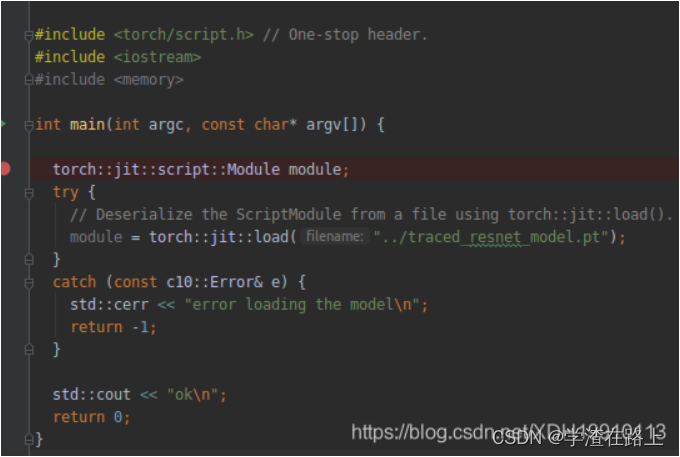### 加载预训练模型

``````import torchvision.models as models
resnet18 = models.resnet18(pretrained=True)     #加载参数
resnet18 = models.resnet18(pretrained=False)    #只加载网络结构，不加载参数``````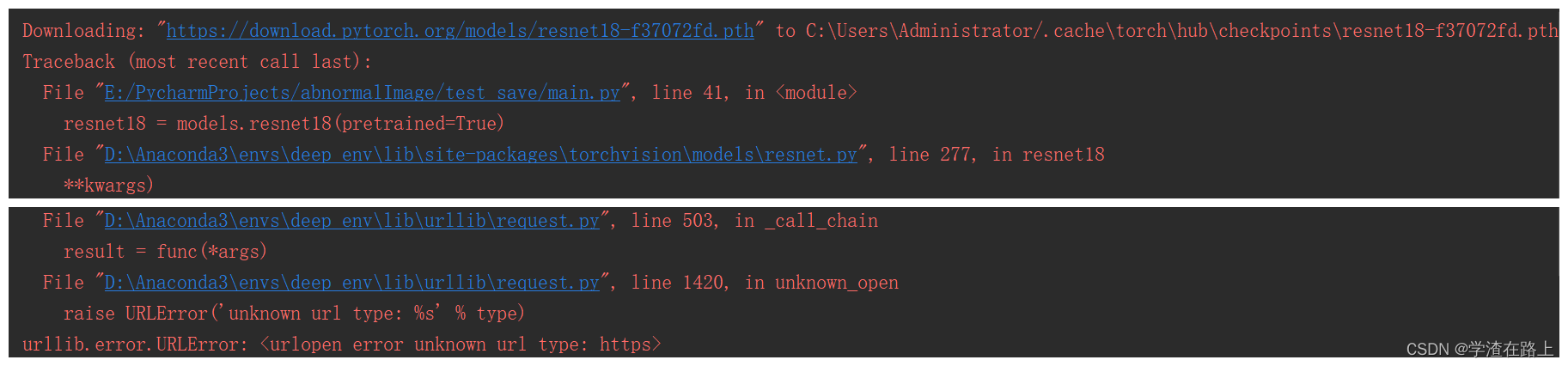``````model_urls = {
}``````

D:\Anaconda3\envs\deep_env\Lib\site-packages\torchvision\models\resnet.py找到上面的地址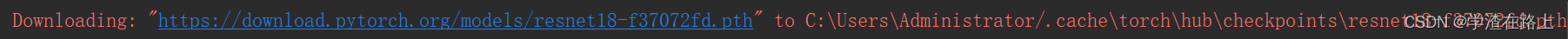`````` resnet50 = models.resnet50(pretrained=True)
pretrained_dict =resnet50.state_dict()
model =Net(...)                        # 读取参数
model_dict = model.state_dict()        # 将pretrained_dict里不属于model_dict的键剔除掉
pretrained_dict = {k: v for k, v in pretrained_dict.items() if k in model_dict}
model_dict.update(pretrained_dict)     # 更新现有的model_dict

``````resnet34 = models.resnet34(pretrained=True)
resnet34.fc = nn.Linear(512, 2)``````

### 保存模型再加载精度损失

pytorch保存模型再加载比训练时低了两个点，这是为什么呢？ – 知乎

``````test_dataloader = torch.utils.data.DataLoader(test_dataset, batch_size=1, shuffle=False,                                  num_workers=args.num_workers)
model.eval()
torch.save(model.state_dict(), 'rrr.pth')``````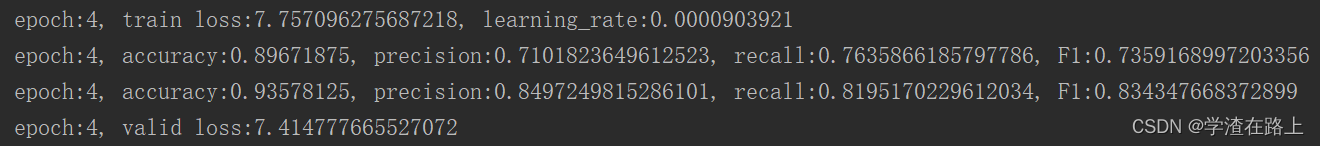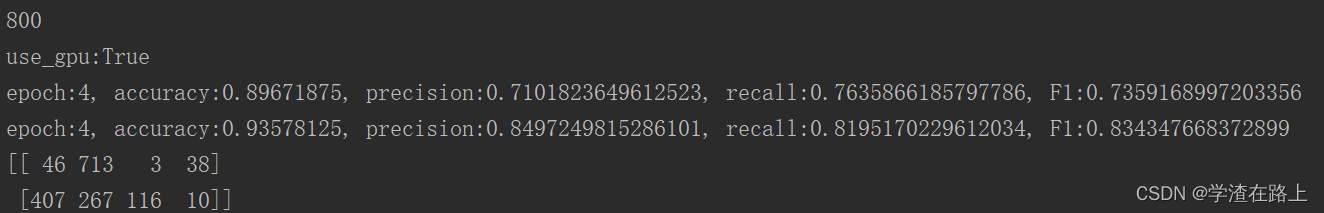``````model.load_state_dict(torch.load(load_pth_name))
model.eval() ``````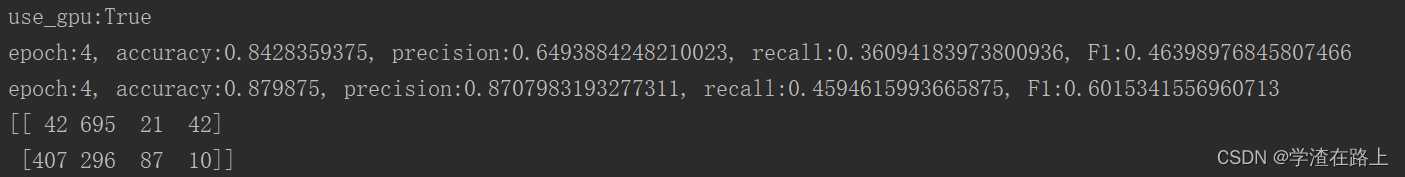### 参考链接

pytorch如何保存模型？ – 知乎

Serialization semantics — PyTorch 1.12 documentation

pytorch模型加载方法汇总 – 走看看

https://www.jianshu.com/p/4905bf8e06e5

PyTorch模型读写、参数初始化、Finetune – leizhao – 博客园

(0)